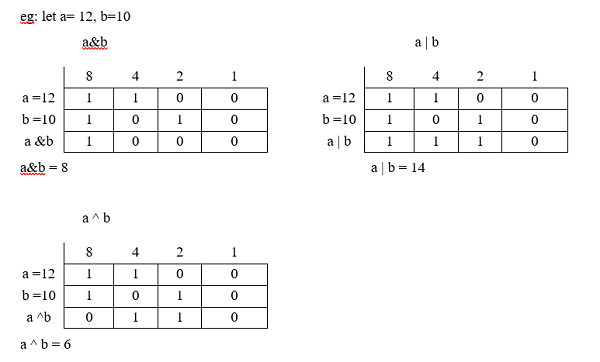# C program for Addition and Multiplication by 2 using Bitwise Operations.

Bitwise operators operate on bits (i.e. on binary values of on operand)

OperatorDescription
&Bitwise AND
|Bitwise OR
^Bitwise XOR
<<Left Shift
>>Right Shift
-One's complement

Bitwise AND
aba & b
000
010
100
111

Bitwise OR
aba | b
000
011
101
111
Bitwise XOR
aba ^ b
000
011
101
110## Example

Following is the C program for addition and multiplication by 2 with the help of bitwise operators −

Live Demo

#include<stdio.h>
main(){
int a;
printf("Enter a");
scanf("%d",&a);
printf("%d*2=%d ",a,a<<1);
printf("%d/2=%d ",a,a>>1);
}

## Output

When the above program is executed, it produces the following output −

Run 1:
Enter a
45
45*2=90
45/2=22
Run 2:
Enter a
65
65*2=130
65/2=32